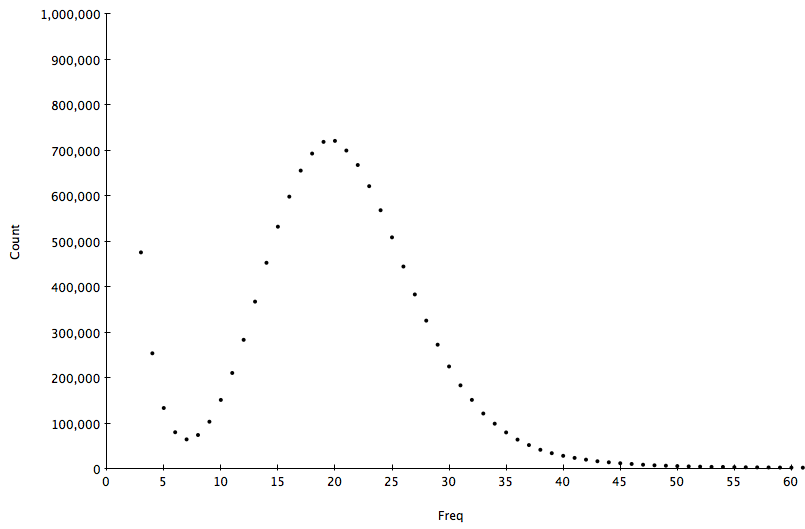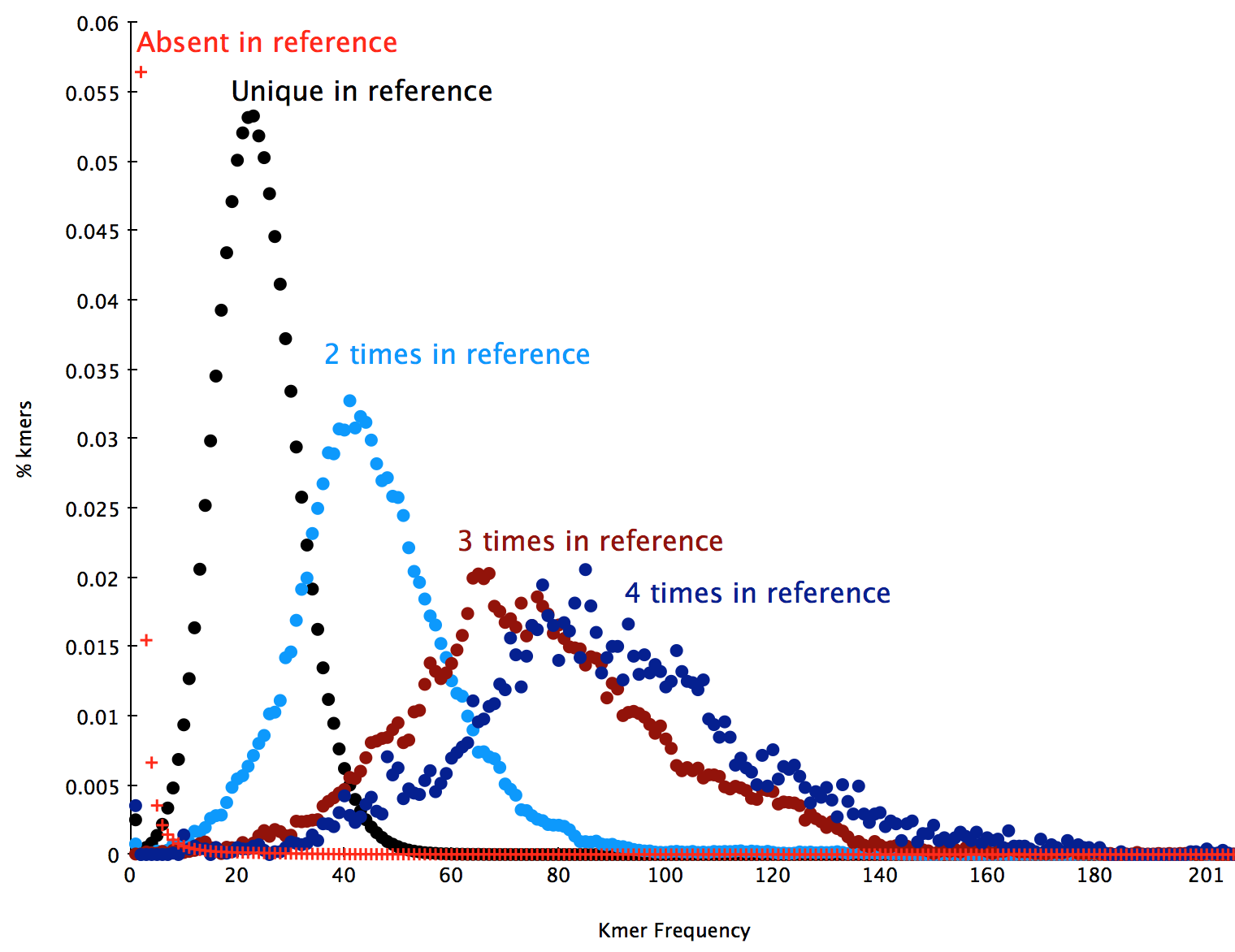3. K-mer spectra¶

A K-mer spectra is a graphical representation of a dataset showing how many short fixed length words (k-mers) appear a certain number of times. The frequency of occurance is plotted on the x-axis and the number of k-mers on the y-axis. The k-mer spectra is composed of distributions representing groups of motifs at different frequencies in the sample, plus biases. Given not too many biases, the shape of the distributions provides a useful set of properties describing the biological sample, the sequencing process and the amount of useful data in the dataset.

A typical 31-mer spectrum of S.cerevisae S288C WGS dataset is shown in the following figure:This is composed of an error component containing a huge amount of rare motifs at frequency < 7 arising from errors in the sequencing process, and a several other components as distributions with different modes according to how many times a motif appears on the genome (once, twice, three times etc.). The following plot shows the decomposition of this distribution into it’s component distributions: